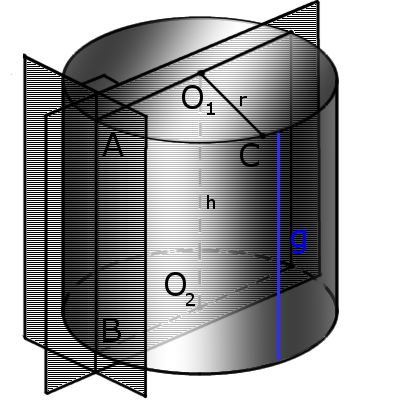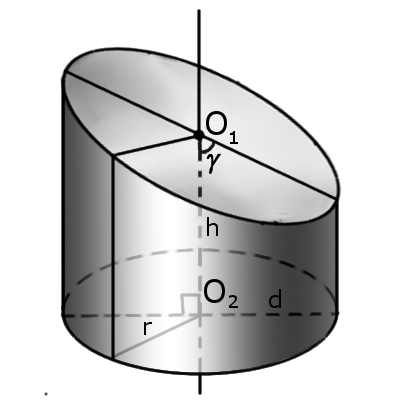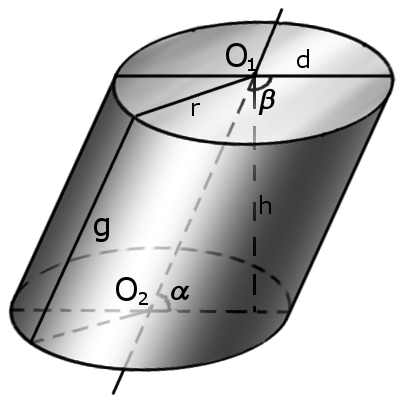Study of mathematics online.
Study math with us and make sure that "Mathematics is easy!"

# Cylinder. Formulas and properties of a cylinder

Definition.
Cylinder is a geometric body that limited cylindrical surface and two planes, called basics of cylinder (top and bottom).
A cylindrical surface is a surface obtained by moving a straight line (generatrix) parallel to itself, along a flat curve.
The cylinder bases are plane figures formed by the intersection of a cylindrical surface with two planes.
Definition. Generatrix of cylinder g is a combination of all segments parallel (AB), which connect the cylinder bases.Fig. 1 Fig. 2 Fig. 3

## Right circular cylinder

Commonly the word cylinder is understood to refer to a finite section of a right circular cylinder having a finite height with circular ends perpendicular to the axis.
Right circular cylinder described as a three-dimensional shape that is formed by rotating of rectangle on its side at 360°. In this case formed a right circular cylinder with a circle at the base of the cylinder.
Definition. Radius of cylinder r of the cylinder is a radius of bases.
Definition. Diameter of cylinder d is a diameter of bases.
Definition. Height of cylinder h is a distance between the bases of the cylinder.
Definition. Axis is a line passing through the centers of the cylinder bases.
Definition. Surface area consisting of side area (lateral area), top area and bottom area.
Definition. Axis section is a section of the cylinder plane passing through the axis of the cylinder.
Definition. Tangent plan to the cylinder is a plane passing through the generatrix of the cylinder and perpendicular to the axial section of the cylinder.
Formula. Volume of a cylinder:
 V = πr2h = π d2 h , 4
where r is a radius of the base, h is height of a cylinder, d is diameter of the base.
Formula. Lateral area (Al) in terms of the radius and height:

Al = 2πrh = πdh

Formula. Surface area (As) in terms of the radius and height:

As = 2πr(h + r)

If the bases (top and bottom surfaces) are not parallel the cylinder called oblique (Fig.2).
If the foundations are not perpendicular to the height the cylinder called slanted (Fig.3).